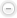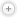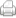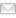##Text SizePrintEmail From: To: Hey! Check out this news page: https://www.thebackgroundinvestigator.com/Articles/Civil-Proceedings-in-Ontario/2801/index.php. \$(document).ready(function() { \$('#Content.int img').each(function() { var subTitle = \$(this).attr('alt'); if(subTitle) { var floatPosition = ''; if(\$(this).hasClass('floatLeft') || (\$(this).css('float')=='left')) { \$(this).removeClass('floatLeft'); floatPosition = ' floatLeft'; } if(\$(this).hasClass('floatRight') || (\$(this).css('float')=='right')) { \$(this).removeClass('floatRight'); floatPosition = ' floatRight'; } \$(this).wrap('<div class="capWrap'+floatPosition+'" />'); \$('.capWrap').last().append('<p>'+subTitle+'</p>'); } }); }); function closeEmailPop() { \$('#email_page').animate({'opacity':0}, 300, function() { \$(this).css('display', 'none'); }); } \$(document).ready(function() { \$('#emailBtn').click(function() { \$('#email_page').css('display', 'block').animate({'opacity':1}, 300); }); \$('#send').click(function() { \$.post('/_includes/send_message.php', \$('#sendPage').serialize(), function(data) { if(data.errors) { \$('#sendPage input').css('border', '1px solid #006699'); for(x in data.errors) { \$('#sendPage input[name="'+data.errors[x]+'"]').css('border','red 1px solid'); } } else { closeEmailPop(); } }, 'json'); }); \$('#cancel').click(function() { closeEmailPop(); }); \$('#minus').click(function() { \$("#Content *").each(function() { var curSize = \$(this).css('font-size'); curSize = curSize.substr(0,2); \$(this).css('font-size',(Number(curSize)-2)+'px'); }); }); \$('#plus').click(function() { \$("#Content *").each(function() { var curSize = \$(this).css('font-size'); curSize = curSize.substr(0,2); \$(this).css('font-size',(Number(curSize)+2)+'px'); }); }); });

January , posted by